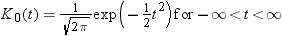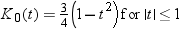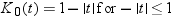# DENSITY Statement

Creates a density curve that shows the distribution of values in your data.
 Interaction: The DENSITY statement can be combined only with the DENSITY and HISTOGRAM statements in the SGPANEL procedure. Examples: About Density Plots

## Syntax

DENSITY response-variable < / option(s)>;

### Summary of Optional Arguments

DENSITY options
specifies the appearance of the density line.
specifies the scaling that is used for the response axis.
specifies the type of distribution curve that is used for the density plot.
Plot options
specifies how many times observations are repeated for computational purposes.
specifies a label that identifies the density plot in the legend.
specifies a name for the plot.
specifies the degree of transparency for the density curve.

### Required Argument

response-variable
specifies the variable for the x axis. The variable must be numeric.

### Optional Arguments

FREQ= numeric-variable
specifies how many times observations are repeated for computational purposes. Each observation is repeated n times for computational purposes, where n is the value of the numeric variable. If n is not an integer, then it is truncated to an integer. If n is less than 1 or missing, then it is excluded from the analysis.
LEGENDLABEL= “text-string
specifies a label that identifies the density plot in the legend. By default, the label identifies the type of density curve. If you specify TYPE=NORMAL, then the default label is “Normal.” If you specify TYPE=KERNEL, then the default label is “Kernel.”
Note:User-specified parameters from the TYPE= option are included in the label by default.
LINEATTRS= style-element <(options)> | (options)
specifies the appearance of the density line. You can specify the appearance by using a style element or by using suboptions. If you specify a style element, you can also specify suboptions to override specific appearance attributes.
options can be one or more of the following:
COLOR= color
specifies the color of the line. You can specify colors using the same color schemes that are supported by SAS/GRAPH software. For more information, see Color-Naming Schemes in SAS/GRAPH: Reference.
Default:The default color is specified by the ContrastColor attribute of the GraphFit style element in the current style.
PATTERN= line-pattern
specifies the line pattern for the line. You can reference SAS patterns by number or by name. For a list of line patterns, see Line Patterns.
Default:The default line pattern is specified by the LineStyle attribute of the GraphFit style element in the current style.
THICKNESS= n <units>
specifies the thickness of the line. You can also specify the unit of measure. The default unit is pixels. For a list of measurement units that are supported, see Units of Measurement.
Default:The default line thickness is specified by the LineThickness attribute of the GraphFit style element in the current style.
NAME= “text-string
specifies a name for the plot. You can use the name to refer to this plot in other statements.
Note:The name is case-sensitive. When you refer to the specified name in other statements, you must use the same exact capitalization.
SCALE= COUNT | DENSITY | PERCENT | PROPORTION
specifies the scaling that is used for the response axis. Specify one of the following values:
COUNT
the axis displays the frequency count.
DENSITY
the axis displays the density estimate values.
PERCENT
the axis displays values as a percentage of the total.
PROPORTION
the axis displays values in proportion to the total.
Default:PERCENT
TRANSPARENCY= numeric-value
specifies the degree of transparency for the density curve. Specify a value from 0.0 (completely opaque) to 1.0 (completely transparent).
Default:0.0
TYPE = NORMAL < (normal-opts)>| KERNEL < (kernel-opts)>
specifies the type of distribution curve that is used for the density plot. Specify one of the following keywords:
NORMAL < (normal-opts)>
specifies a normal density estimate, with a mean and a standard deviation.
normal-opts can be one or more of the following values:
MU=numeric-value
specifies the mean value that is used in the density function equation. By default, the mean value is calculated from the data.
SIGMA=numeric-value
specifies the standard deviation value that is used in the density function equation. The value that you specify for the SIGMA= suboption must be a positive number. By default, the standard deviation value is calculated from the data.
KERNEL < (kernel-opts)>
specifies a nonparametric kernel density estimate.
kernel-opts can be:
C= numeric-value
specifies the standardized bandwidth for a number that is greater than 0 and less than or equal to 100.
The value that you specify for the C= suboption affects the value of λ as shown in the following equation: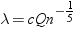In this equation c is the standardized bandwidth, Q is the interquartile range, and n is the sample size.
WEIGHT= NORMAL | QUADRATIC | TRIANGULAR
specifies the weight function. You can specify either normal, quadratic, or triangular weight function.
Default:NORMAL
Default:NORMAL

## Normal Density Function

When the type of the density curve is NORMAL, the fitted density function equation is as follows.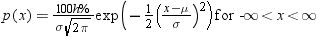In the equation, μ is the mean, and σ is the standard deviation. You can specify μ by using the MU= suboption and σ by using the SIGMA= suboption.

## Kernel Density Function

When the TYPE of the density curve is KERNEL, the general form of the kernel density estimator is as follows.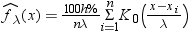In the equation,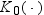is the weight function, λ is the bandwidth, n is the sample size, and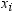is the ith observation. You can use the C= suboption to specify the bandwidth and the WEIGHT= suboption to specify the weight function.

## Kernel Density Weight Functions

The formulas for the weight functions are as follows.
NORMAL